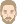## Moisture Storage Function - 3 point formula

marco.larcher
WUFI UserPosts: 3
Joined: Thu May 13, 2021 2:52 am -1100

### Moisture Storage Function - 3 point formula

Dear IBP,

typically moisture moisture storage function is based on few measured values (0.65, 0.80, 0.93, 0.97, free saturation) while the rest of the points are interpolated (0.99, 0.995, 0.999, 0.9995, 0.9999).
According to the "Material Information" I see that a 3 point formula is used.

I searched the web for a 3 point interpolation method but I failed to identify the one that reproduces the WUFI sorption isotherms.

Could you be more specific on the interpolation methodology that is applied (is there an analytical formula that is used for interpolation?) or indicate literature where to collect more information?

Best Regards
Marco

ThomasPosts: 365
Joined: Sun Jun 19, 2005 10:33 pm -1100

### Re: Moisture Storage Function - 3 point formula

Hi Marco,

the 3-point formula has been introduced by Dr. A. Holm in his dissertation

Holm A.:
Ermittlung der Genauigkeit von instationären hygrothermischen Bauteilberechnungen mittels eines stochastischen Konzeptes,
Dissertation Uni Stuttgart, 2001 (in German)
https://publica.fraunhofer.de/entities/ ... cd/details
(See Formula (10) and the red dotted lines in Figures 19, 20, 21)

and

Holm A., Krus M., Künzel H.M.:
Approximation der Feuchtespeicherfunktion aus einfach bestimmbaren Kennwerten
IBP-Mitteilung 406, 2002
https://publica.fraunhofer.de/entities/ ... 90/details

$$u(p_c) = \frac{u_f}{1 + \left(\frac{p_c}{pk1}\right)^{pk2}}$$
$$u(p_c)$$ water content as function of the capillary pressure $$p_c$$, kg/m³
$$u_f$$: water content at free saturation, kg/m³
$$p_c$$: capillary pressure, Pa
$$pk1, pk2$$: fit parameters

Holm A., Kuenzel H.M.:
Uncertainty Approaches for Hygrothermal Building Simulations - Drying of an AAC Flat Roof in Different Climates

Seventh International IBPSA Conference
Rio de Janeiro, Brazil
August 13-15, 2001
https://wufi.de/literatur/Holm,%20K%C3% ... ilding.pdf

The function has the shape of a "rounded step" function and thus reproduces the typical shape which a moisture storage curve has when the water content is plotted against the logarithm of the capillary pressure.

It's called "3-point formula" in WUFI to distinguish it from the "two-point" formula which WUFI uses for approximating the moisture storage function if two points (usually u80 and uf) are given. The 3-point formula is more flexible, but it needs three points as input since there are three free parameters ($$u_f$$, $$pk1$$, $$pk2$$). We usually choose the points u(RH=80%), u(RH=95 %) and u_f, but other choices are possible as well. If more than three points of the moisture storage function are available we usually fit the curve to all of them in a least-squares fit:Three-point-formula _OSB 650 _ uf = 470 kgm3 _ B.png (28.26 KiB) Viewed 72 times
Best regards,
Thomas

Keywords: 3-point formula, 3-point function, 3-Punkte-Formel, 3-Punkte-Funktion, drei-Punkte-Funktion, drei-Punkte-Formel